Home » A Comprehensive Guide to Understand and Implement Text Classification in Python

# A Comprehensive Guide to Understand and Implement Text Classification in Python

## Introduction

One of the widely used natural language processing task in different business problems is “Text Classification”. The goal of text classification is to automatically classify the text documents into one or more defined categories. Some examples of text classification are:

• Understanding audience sentiment from social media,
• Detection of spam and non-spam emails,
• Auto tagging of customer queries, and
• Categorization of news articles into defined topics.

If you’re a beginner in NLP, then you’ve come to the right place! We have designed a comprehensive NLP course just for you. It is one of our most popular courses and is just the right guide to kick start your NLP journey.

## Problem Statement

The objective of this task is to detect hate speech in tweets. For the sake of simplicity, we say a tweet contains hate speech if it has a racist or sexist sentiment associated with it. So, the task is to classify racist or sexist tweets from other tweets.

Formally, given a training sample of tweets and labels, where label ‘1’ denotes the tweet is racist/sexist and label ‘0’ denotes the tweet is not racist/sexist, your objective is to predict the labels on the test dataset.

Practice Now

In this article, I will explain about the text classification and the step by step process to implement it in python.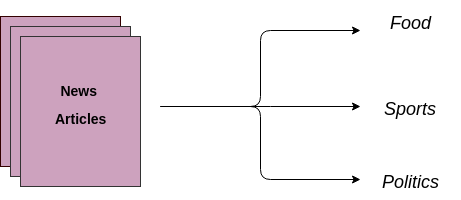Text Classification is an example of supervised machine learning task since a labelled dataset containing text documents and their labels is used for train a classifier. An end-to-end text classification pipeline is composed of three main components:

1. Dataset Preparation: The first step is the Dataset Preparation step which includes the process of loading a dataset and performing basic pre-processing. The dataset is then splitted into train and validation sets.
2. Feature Engineering: The next step is the Feature Engineering in which the raw dataset is transformed into flat features which can be used in a machine learning model. This step also includes the process of creating new features from the existing data.
3. Model Training: The final step is the Model Building step in which a machine learning model is trained on a labelled dataset.

4. Improve Performance of Text Classifier: In this article, we will also look at the different ways to improve the performance of text classifiers.

Note : This article does not narrate NLP tasks in depth. If you want to revise the basics and come back here, you can always go through this article.

Lets implement basic components in a step by step manner in order to create a text classification framework in python. To start with, import all the required libraries.

You would need requisite libraries to run this code – you can install them at their individual official links

# libraries for dataset preparation, feature engineering, model training
```from sklearn import model_selection, preprocessing, linear_model, naive_bayes, metrics, svm
from sklearn.feature_extraction.text import TfidfVectorizer, CountVectorizer
from sklearn import decomposition, ensemble

import pandas, xgboost, numpy, textblob, string
from keras.preprocessing import text, sequence
from keras import layers, models, optimizers
```

## 1. Dataset preparation

For the purpose of this article, I am the using dataset of amazon reviews which can be downloaded at this link. The dataset consists of 3.6M text reviews and their labels, we will use only a small fraction of data. To prepare the dataset, load the downloaded data into a pandas dataframe containing two columns – text and label. (Source)

```# load the dataset
labels, texts = [], []
for i, line in enumerate(data.split("\n")):
content = line.split()
labels.append(content)
texts.append(" ".join(content[1:]))

# create a dataframe using texts and lables
trainDF = pandas.DataFrame()
trainDF['text'] = texts
trainDF['label'] = labels
```

Next, we will split the dataset into training and validation sets so that we can train and test classifier. Also, we will encode our target column so that it can be used in machine learning models.

```# split the dataset into training and validation datasets
train_x, valid_x, train_y, valid_y = model_selection.train_test_split(trainDF['text'], trainDF['label'])

# label encode the target variable
encoder = preprocessing.LabelEncoder()
train_y = encoder.fit_transform(train_y)
valid_y = encoder.fit_transform(valid_y)
```

## 2. Feature Engineering

The next step is the feature engineering step. In this step, raw text data will be transformed into feature vectors and new features will be created using the existing dataset. We will implement the following different ideas in order to obtain relevant features from our dataset.

2.1 Count Vectors as features
2.2 TF-IDF Vectors as features

• Word level
• N-Gram level
• Character level

2.3 Word Embeddings as features
2.4 Text / NLP based features
2.5 Topic Models as features

Lets look at the implementation of these ideas in detail.

### 2.1 Count Vectors as features

Count Vector is a matrix notation of the dataset in which every row represents a document from the corpus, every column represents a term from the corpus, and every cell represents the frequency count of a particular term in a particular document.

```# create a count vectorizer object
count_vect = CountVectorizer(analyzer='word', token_pattern=r'\w{1,}')
count_vect.fit(trainDF['text'])

# transform the training and validation data using count vectorizer object
xtrain_count =  count_vect.transform(train_x)
xvalid_count =  count_vect.transform(valid_x)
```

### 2.2 TF-IDF Vectors as features

TF-IDF score represents the relative importance of a term in the document and the entire corpus. TF-IDF score is composed by two terms: the first computes the normalized Term Frequency (TF), the second term is the Inverse Document Frequency (IDF), computed as the logarithm of the number of the documents in the corpus divided by the number of documents where the specific term appears.

TF(t) = (Number of times term t appears in a document) / (Total number of terms in the document)
IDF(t) = log_e(Total number of documents / Number of documents with term t in it)

TF-IDF Vectors can be generated at different levels of input tokens (words, characters, n-grams)

a. Word Level TF-IDF : Matrix representing tf-idf scores of every term in different documents
b. N-gram Level TF-IDF : N-grams are the combination of N terms together. This Matrix representing tf-idf scores of N-grams
c. Character Level TF-IDF : Matrix representing tf-idf scores of character level n-grams in the corpus

```# word level tf-idf
tfidf_vect = TfidfVectorizer(analyzer='word', token_pattern=r'\w{1,}', max_features=5000)
tfidf_vect.fit(trainDF['text'])
xtrain_tfidf =  tfidf_vect.transform(train_x)
xvalid_tfidf =  tfidf_vect.transform(valid_x)

# ngram level tf-idf
tfidf_vect_ngram = TfidfVectorizer(analyzer='word', token_pattern=r'\w{1,}', ngram_range=(2,3), max_features=5000)
tfidf_vect_ngram.fit(trainDF['text'])
xtrain_tfidf_ngram =  tfidf_vect_ngram.transform(train_x)
xvalid_tfidf_ngram =  tfidf_vect_ngram.transform(valid_x)

# characters level tf-idf
tfidf_vect_ngram_chars = TfidfVectorizer(analyzer='char', token_pattern=r'\w{1,}', ngram_range=(2,3), max_features=5000)
tfidf_vect_ngram_chars.fit(trainDF['text'])
xtrain_tfidf_ngram_chars =  tfidf_vect_ngram_chars.transform(train_x)
xvalid_tfidf_ngram_chars =  tfidf_vect_ngram_chars.transform(valid_x)
```

### 2.3 Word Embeddings

A word embedding is a form of representing words and documents using a dense vector representation. The position of a word within the vector space is learned from text and is based on the words that surround the word when it is used. Word embeddings can be trained using the input corpus itself or can be generated using pre-trained word embeddings such as Glove, FastText, and Word2Vec. Any one of them can be downloaded and used as transfer learning. One can read more about word embeddings here.

Following snnipet shows how to use pre-trained word embeddings in the model. There are four essential steps:

2. Creating a tokenizer object
3. Transforming text documents to sequence of tokens and pad them
4. Create a mapping of token and their respective embeddings

```# load the pre-trained word-embedding vectors
embeddings_index = {}
for i, line in enumerate(open('data/wiki-news-300d-1M.vec')):
values = line.split()
embeddings_index[values] = numpy.asarray(values[1:], dtype='float32')

# create a tokenizer
token = text.Tokenizer()
token.fit_on_texts(trainDF['text'])
word_index = token.word_index

# convert text to sequence of tokens and pad them to ensure equal length vectors

# create token-embedding mapping
embedding_matrix = numpy.zeros((len(word_index) + 1, 300))
for word, i in word_index.items():
embedding_vector = embeddings_index.get(word)
if embedding_vector is not None:
embedding_matrix[i] = embedding_vector
```

### 2.4 Text / NLP based features

A number of extra text based features can also be created which sometimes are helpful for improving text classification models. Some examples are:

1. Word Count of the documents – total number of words in the documents
2. Character Count of the documents – total number of characters in the documents
3. Average Word Density of the documents – average length of the words used in the documents
4. Puncutation Count in the Complete Essay – total number of punctuation marks in the documents
5. Upper Case Count in the Complete Essay – total number of upper count words in the documents
6. Title Word Count in the Complete Essay – total number of proper case (title) words in the documents
7. Frequency distribution of Part of Speech Tags:
• Noun Count
• Verb Count
• Pronoun Count

These features are highly experimental ones and should be used according to the problem statement only.

```trainDF['char_count'] = trainDF['text'].apply(len)
trainDF['word_count'] = trainDF['text'].apply(lambda x: len(x.split()))
trainDF['word_density'] = trainDF['char_count'] / (trainDF['word_count']+1)
trainDF['punctuation_count'] = trainDF['text'].apply(lambda x: len("".join(_ for _ in x if _ in string.punctuation)))
trainDF['title_word_count'] = trainDF['text'].apply(lambda x: len([wrd for wrd in x.split() if wrd.istitle()]))
trainDF['upper_case_word_count'] = trainDF['text'].apply(lambda x: len([wrd for wrd in x.split() if wrd.isupper()]))
```
```pos_family = {
'noun' : ['NN','NNS','NNP','NNPS'],
'pron' : ['PRP','PRP\$','WP','WP\$'],
'verb' : ['VB','VBD','VBG','VBN','VBP','VBZ'],
}

# function to check and get the part of speech tag count of a words in a given sentence
def check_pos_tag(x, flag):
cnt = 0
try:
wiki = textblob.TextBlob(x)
for tup in wiki.tags:
ppo = list(tup)
if ppo in pos_family[flag]:
cnt += 1
except:
pass
return cnt

trainDF['noun_count'] = trainDF['text'].apply(lambda x: check_pos_tag(x, 'noun'))
trainDF['verb_count'] = trainDF['text'].apply(lambda x: check_pos_tag(x, 'verb'))
trainDF['pron_count'] = trainDF['text'].apply(lambda x: check_pos_tag(x, 'pron'))
```

### 2.5 Topic Models as features

Topic Modelling is a technique to identify the groups of words (called a topic) from a collection of documents that contains best information in the collection. I have used Latent Dirichlet Allocation for generating Topic Modelling Features. LDA is an iterative model which starts from a fixed number of topics. Each topic is represented as a distribution over words, and each document is then represented as a distribution over topics. Although the tokens themselves are meaningless, the probability distributions over words provided by the topics provide a sense of the different ideas contained in the documents. One can read more about topic modelling here

Lets see its implementation:

```# train a LDA Model
lda_model = decomposition.LatentDirichletAllocation(n_components=20, learning_method='online', max_iter=20)
X_topics = lda_model.fit_transform(xtrain_count)
topic_word = lda_model.components_
vocab = count_vect.get_feature_names()

# view the topic models
n_top_words = 10
topic_summaries = []
for i, topic_dist in enumerate(topic_word):
topic_words = numpy.array(vocab)[numpy.argsort(topic_dist)][:-(n_top_words+1):-1]
topic_summaries.append(' '.join(topic_words))
```

## 3. Model Building

The final step in the text classification framework is to train a classifier using the features created in the previous step. There are many different choices of machine learning models which can be used to train a final model. We will implement following different classifiers for this purpose:

1. Naive Bayes Classifier
2. Linear Classifier
3. Support Vector Machine
4. Bagging Models
5. Boosting Models
6. Shallow Neural Networks
7. Deep Neural Networks
• Convolutional Neural Network (CNN)
• Long Short Term Modelr (LSTM)
• Gated Recurrent Unit (GRU)
• Bidirectional RNN
• Recurrent Convolutional Neural Network (RCNN)
• Other Variants of Deep Neural Networks

Lets implement these models and understand their details. The following function is a utility function which can be used to train a model. It accepts the classifier, feature_vector of training data, labels of training data and feature vectors of valid data as inputs. Using these inputs, the model is trained and accuracy score is computed.

```def train_model(classifier, feature_vector_train, label, feature_vector_valid, is_neural_net=False):
# fit the training dataset on the classifier
classifier.fit(feature_vector_train, label)

# predict the labels on validation dataset
predictions = classifier.predict(feature_vector_valid)

if is_neural_net:
predictions = predictions.argmax(axis=-1)

return metrics.accuracy_score(predictions, valid_y)
```

### 3.1 Naive Bayes

Implementing a naive bayes model using sklearn implementation with different features

Naive Bayes is a classification technique based on Bayes’ Theorem with an assumption of independence among predictors. A Naive Bayes classifier assumes that the presence of a particular feature in a class is unrelated to the presence of any other feature here .

```# Naive Bayes on Count Vectors
accuracy = train_model(naive_bayes.MultinomialNB(), xtrain_count, train_y, xvalid_count)
print "NB, Count Vectors: ", accuracy

# Naive Bayes on Word Level TF IDF Vectors
accuracy = train_model(naive_bayes.MultinomialNB(), xtrain_tfidf, train_y, xvalid_tfidf)
print "NB, WordLevel TF-IDF: ", accuracy

# Naive Bayes on Ngram Level TF IDF Vectors
accuracy = train_model(naive_bayes.MultinomialNB(), xtrain_tfidf_ngram, train_y, xvalid_tfidf_ngram)
print "NB, N-Gram Vectors: ", accuracy

# Naive Bayes on Character Level TF IDF Vectors
accuracy = train_model(naive_bayes.MultinomialNB(), xtrain_tfidf_ngram_chars, train_y, xvalid_tfidf_ngram_chars)
print "NB, CharLevel Vectors: ", accuracy
```
```NB, Count Vectors:  0.7004
NB, WordLevel TF-IDF:  0.7024
NB, N-Gram Vectors:  0.5344
NB, CharLevel Vectors:  0.6872
```

### 3.2 Linear Classifier

Implementing a Linear Classifier (Logistic Regression)

Logistic regression measures the relationship between the categorical dependent variable and one or more independent variables by estimating probabilities using a logistic/sigmoid function. One can read more about logistic regression here

```# Linear Classifier on Count Vectors
accuracy = train_model(linear_model.LogisticRegression(), xtrain_count, train_y, xvalid_count)
print "LR, Count Vectors: ", accuracy

# Linear Classifier on Word Level TF IDF Vectors
accuracy = train_model(linear_model.LogisticRegression(), xtrain_tfidf, train_y, xvalid_tfidf)
print "LR, WordLevel TF-IDF: ", accuracy

# Linear Classifier on Ngram Level TF IDF Vectors
accuracy = train_model(linear_model.LogisticRegression(), xtrain_tfidf_ngram, train_y, xvalid_tfidf_ngram)
print "LR, N-Gram Vectors: ", accuracy

# Linear Classifier on Character Level TF IDF Vectors
accuracy = train_model(linear_model.LogisticRegression(), xtrain_tfidf_ngram_chars, train_y, xvalid_tfidf_ngram_chars)
print "LR, CharLevel Vectors: ", accuracy
```
```LR, Count Vectors:  0.7048
LR, WordLevel TF-IDF:  0.7056
LR, N-Gram Vectors:  0.4896
LR, CharLevel Vectors:  0.7012
```

### 3.3 Implementing a SVM Model

Support Vector Machine (SVM) is a supervised machine learning algorithm which can be used for both classification or regression challenges. The model extracts a best possible hyper-plane / line that segregates the two classes. One can read more about it here

```# SVM on Ngram Level TF IDF Vectors
accuracy = train_model(svm.SVC(), xtrain_tfidf_ngram, train_y, xvalid_tfidf_ngram)
print "SVM, N-Gram Vectors: ", accuracy
```
```SVM, N-Gram Vectors:  0.5296
```

### 3.4 Bagging Model

Implementing a Random Forest Model

Random Forest models are a type of ensemble models, particularly bagging models. They are part of the tree based model family. One can read more about Bagging and random forests here

```# RF on Count Vectors
accuracy = train_model(ensemble.RandomForestClassifier(), xtrain_count, train_y, xvalid_count)
print "RF, Count Vectors: ", accuracy

# RF on Word Level TF IDF Vectors
accuracy = train_model(ensemble.RandomForestClassifier(), xtrain_tfidf, train_y, xvalid_tfidf)
print "RF, WordLevel TF-IDF: ", accuracy
```
```RF, Count Vectors:  0.6972
RF, WordLevel TF-IDF:  0.6988
```

### 3.5 Boosting Model

Boosting models are another type of ensemble models part of tree based models. Boosting is a machine learning ensemble meta-algorithm for primarily reducing bias, and also variance in supervised learning, and a family of machine learning algorithms that convert weak learners to strong ones. A weak learner is defined to be a classifier that is only slightly correlated with the true classification (it can label examples better than random guessing). Read more about these models here

```# Extereme Gradient Boosting on Count Vectors
accuracy = train_model(xgboost.XGBClassifier(), xtrain_count.tocsc(), train_y, xvalid_count.tocsc())
print "Xgb, Count Vectors: ", accuracy

# Extereme Gradient Boosting on Word Level TF IDF Vectors
accuracy = train_model(xgboost.XGBClassifier(), xtrain_tfidf.tocsc(), train_y, xvalid_tfidf.tocsc())
print "Xgb, WordLevel TF-IDF: ", accuracy

# Extereme Gradient Boosting on Character Level TF IDF Vectors
accuracy = train_model(xgboost.XGBClassifier(), xtrain_tfidf_ngram_chars.tocsc(), train_y, xvalid_tfidf_ngram_chars.tocsc())
print "Xgb, CharLevel Vectors: ", accuracy
```
```/usr/local/lib/python2.7/dist-packages/sklearn/preprocessing/label.py:151: DeprecationWarning: The truth value of an empty array is ambiguous. Returning False, but in future this will result in an error. Use `array.size > 0` to check that an array is not empty.
if diff:
/usr/local/lib/python2.7/dist-packages/sklearn/preprocessing/label.py:151: DeprecationWarning: The truth value of an empty array is ambiguous. Returning False, but in future this will result in an error. Use `array.size > 0` to check that an array is not empty.
if diff:
```
```Xgb, Count Vectors:  0.6324
Xgb, WordLevel TF-IDF:  0.6364
Xgb, CharLevel Vectors:  0.6548
```
```/usr/local/lib/python2.7/dist-packages/sklearn/preprocessing/label.py:151: DeprecationWarning: The truth value of an empty array is ambiguous. Returning False, but in future this will result in an error. Use `array.size > 0` to check that an array is not empty.
if diff:
```

### 3.6 Shallow Neural Networks

A neural network is a mathematical model that is designed to behave similar to biological neurons and nervous system. These models are used to recognize complex patterns and relationships that exists within a labelled data. A shallow neural network contains mainly three types of layers – input layer, hidden layer, and output layer. Read more about neural networks here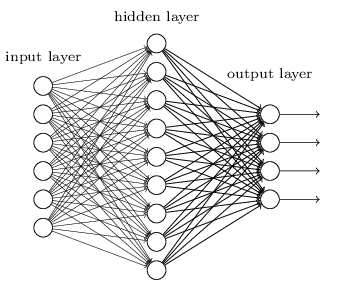```def create_model_architecture(input_size):
# create input layer
input_layer = layers.Input((input_size, ), sparse=True)

# create hidden layer
hidden_layer = layers.Dense(100, activation="relu")(input_layer)

# create output layer
output_layer = layers.Dense(1, activation="sigmoid")(hidden_layer)

classifier = models.Model(inputs = input_layer, outputs = output_layer)
return classifier

classifier = create_model_architecture(xtrain_tfidf_ngram.shape)
accuracy = train_model(classifier, xtrain_tfidf_ngram, train_y, xvalid_tfidf_ngram, is_neural_net=True)
print "NN, Ngram Level TF IDF Vectors",  accuracy
```
```Epoch 1/1
7500/7500 [==============================] - 1s 67us/step - loss: 0.6909
NN, Ngram Level TF IDF Vectors 0.5296
```

### 3.7 Deep Neural Networks

Deep Neural Networks are more complex neural networks in which the hidden layers performs much more complex operations than simple sigmoid or relu activations. Different types of deep learning models can be applied in text classification problems.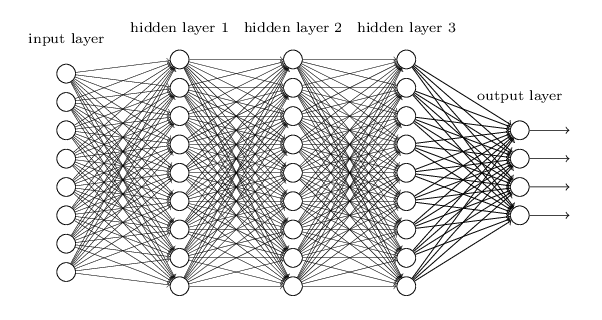#### 3.7.1 Convolutional Neural Network

In Convolutional neural networks, convolutions over the input layer are used to compute the output. This results in local connections, where each region of the input is connected to a neuron in the output. Each layer applies different filters and combines their results.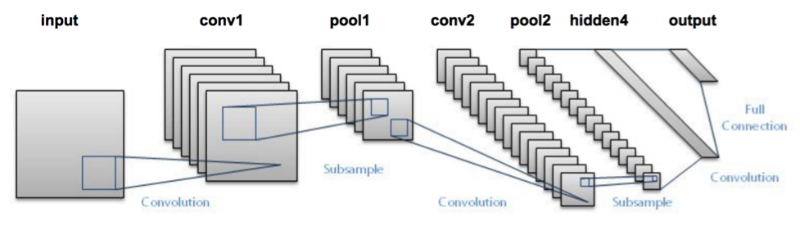```def create_cnn():
input_layer = layers.Input((70, ))

# Add the word embedding Layer
embedding_layer = layers.Embedding(len(word_index) + 1, 300, weights=[embedding_matrix], trainable=False)(input_layer)
embedding_layer = layers.SpatialDropout1D(0.3)(embedding_layer)

conv_layer = layers.Convolution1D(100, 3, activation="relu")(embedding_layer)

pooling_layer = layers.GlobalMaxPool1D()(conv_layer)

output_layer1 = layers.Dense(50, activation="relu")(pooling_layer)
output_layer1 = layers.Dropout(0.25)(output_layer1)
output_layer2 = layers.Dense(1, activation="sigmoid")(output_layer1)

# Compile the model
model = models.Model(inputs=input_layer, outputs=output_layer2)

return model

classifier = create_cnn()
accuracy = train_model(classifier, train_seq_x, train_y, valid_seq_x, is_neural_net=True)
print "CNN, Word Embeddings",  accuracy
```
```Epoch 1/1
7500/7500 [==============================] - 12s 2ms/step - loss: 0.5847
CNN, Word Embeddings 0.5296
```

#### 3.7.2 Recurrent Neural Network – LSTM

Unlike Feed-forward neural networks in which activation outputs are propagated only in one direction, the activation outputs from neurons propagate in both directions (from inputs to outputs and from outputs to inputs) in Recurrent Neural Networks. This creates loops in the neural network architecture which acts as a ‘memory state’ of the neurons. This state allows the neurons an ability to remember what have been learned so far.

The memory state in RNNs gives an advantage over traditional neural networks but a problem called Vanishing Gradient is associated with them. In this problem, while learning with a large number of layers, it becomes really hard for the network to learn and tune the parameters of the earlier layers. To address this problem, A new type of RNNs called LSTMs (Long Short Term Memory) Models have been developed.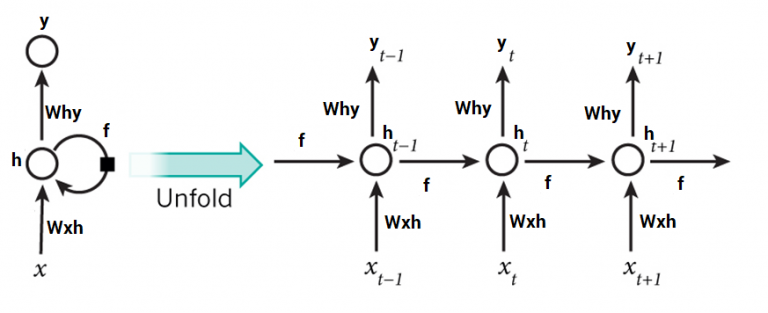def create_rnn_lstm():
```    # Add an Input Layer
input_layer = layers.Input((70, ))

# Add the word embedding Layer
embedding_layer = layers.Embedding(len(word_index) + 1, 300, weights=[embedding_matrix], trainable=False)(input_layer)
embedding_layer = layers.SpatialDropout1D(0.3)(embedding_layer)

lstm_layer = layers.LSTM(100)(embedding_layer)

output_layer1 = layers.Dense(50, activation="relu")(lstm_layer)
output_layer1 = layers.Dropout(0.25)(output_layer1)
output_layer2 = layers.Dense(1, activation="sigmoid")(output_layer1)

# Compile the model
model = models.Model(inputs=input_layer, outputs=output_layer2)

return model

classifier = create_rnn_lstm()
accuracy = train_model(classifier, train_seq_x, train_y, valid_seq_x, is_neural_net=True)
print "RNN-LSTM, Word Embeddings",  accuracy
```
```Epoch 1/1
7500/7500 [==============================] - 22s 3ms/step - loss: 0.6899
RNN-LSTM, Word Embeddings 0.5124
```

#### 3.7.3 Recurrent Neural Network – GRU

Gated Recurrent Units are another form of recurrent neural networks. Lets add a layer of GRU instead of LSTM in our network.

def create_rnn_gru():
```    # Add an Input Layer
input_layer = layers.Input((70, ))

# Add the word embedding Layer
embedding_layer = layers.Embedding(len(word_index) + 1, 300, weights=[embedding_matrix], trainable=False)(input_layer)
embedding_layer = layers.SpatialDropout1D(0.3)(embedding_layer)

lstm_layer = layers.GRU(100)(embedding_layer)

output_layer1 = layers.Dense(50, activation="relu")(lstm_layer)
output_layer1 = layers.Dropout(0.25)(output_layer1)
output_layer2 = layers.Dense(1, activation="sigmoid")(output_layer1)

# Compile the model
model = models.Model(inputs=input_layer, outputs=output_layer2)

return model

classifier = create_rnn_gru()
accuracy = train_model(classifier, train_seq_x, train_y, valid_seq_x, is_neural_net=True)
print "RNN-GRU, Word Embeddings",  accuracy
```
```Epoch 1/1
7500/7500 [==============================] - 19s 3ms/step - loss: 0.6898
RNN-GRU, Word Embeddings 0.5124
```

#### 3.7.4 Bidirectional RNN

RNN layers can be wrapped in Bidirectional layers as well. Lets wrap our GRU layer in bidirectional layer.

def create_bidirectional_rnn():
```    # Add an Input Layer
input_layer = layers.Input((70, ))

# Add the word embedding Layer
embedding_layer = layers.Embedding(len(word_index) + 1, 300, weights=[embedding_matrix], trainable=False)(input_layer)
embedding_layer = layers.SpatialDropout1D(0.3)(embedding_layer)

lstm_layer = layers.Bidirectional(layers.GRU(100))(embedding_layer)

output_layer1 = layers.Dense(50, activation="relu")(lstm_layer)
output_layer1 = layers.Dropout(0.25)(output_layer1)
output_layer2 = layers.Dense(1, activation="sigmoid")(output_layer1)

# Compile the model
model = models.Model(inputs=input_layer, outputs=output_layer2)

return model

classifier = create_bidirectional_rnn()
accuracy = train_model(classifier, train_seq_x, train_y, valid_seq_x, is_neural_net=True)
print "RNN-Bidirectional, Word Embeddings",  accuracy
```
```Epoch 1/1
7500/7500 [==============================] - 32s 4ms/step - loss: 0.6889
RNN-Bidirectional, Word Embeddings 0.5124
```

#### 3.7.5 Recurrent Convolutional Neural Network

Once the essential architectures have been tried out, one can try different variants of these layers such as recurrent convolutional neural network. Another variants can be:

1. Hierarichial Attention Networks
2. Sequence to Sequence Models with Attention
3. Bidirectional Recurrent Convolutional Neural Networks
4. CNNs and RNNs with more number of layers
def create_rcnn():
```    # Add an Input Layer
input_layer = layers.Input((70, ))

# Add the word embedding Layer
embedding_layer = layers.Embedding(len(word_index) + 1, 300, weights=[embedding_matrix], trainable=False)(input_layer)
embedding_layer = layers.SpatialDropout1D(0.3)(embedding_layer)

rnn_layer = layers.Bidirectional(layers.GRU(50, return_sequences=True))(embedding_layer)

conv_layer = layers.Convolution1D(100, 3, activation="relu")(embedding_layer)

pooling_layer = layers.GlobalMaxPool1D()(conv_layer)

output_layer1 = layers.Dense(50, activation="relu")(pooling_layer)
output_layer1 = layers.Dropout(0.25)(output_layer1)
output_layer2 = layers.Dense(1, activation="sigmoid")(output_layer1)

# Compile the model
model = models.Model(inputs=input_layer, outputs=output_layer2)

return model

classifier = create_rcnn()
accuracy = train_model(classifier, train_seq_x, train_y, valid_seq_x, is_neural_net=True)
print "CNN, Word Embeddings",  accuracy
```
```Epoch 1/1
7500/7500 [==============================] - 11s 1ms/step - loss: 0.6902
CNN, Word Embeddings 0.5124
```

## Improving Text Classification Models

While the above framework can be applied to a number of text classification problems, but to achieve a good accuracy some improvements can be done in the overall framework. For example, following are some tips to improve the performance of text classification models and this framework.

1. Text Cleaning : text cleaning can help to reducue the noise present in text data in the form of stopwords, punctuations marks, suffix variations etc. This article can help to understand how to implement text classification in detail.

2. Hstacking Text / NLP features with text feature vectors : In the feature engineering section, we generated a number of different feature vectros, combining them together can help to improve the accuracy of the classifier.

3. Hyperparamter Tuning in modelling : Tuning the paramters is an important step, a number of parameters such as tree length, leafs, network paramters etc can be fine tuned to get a best fit model.

4. Ensemble Models : Stacking different models and blending their outputs can help to further improve the results. Read more about ensemble models here

## Projects

Now, its time to take the plunge and actually play with some other real datasets. So are you ready to take on the challenge? Accelerate your NLP journey with the following Practice Problems:

## End Notes

In this article, we discussed about how to prepare a text dataset like cleaning/creating training and validation dataset, perform different types of feature engineering like Count Vector/TF-IDF/ Word Embedding/ Topic Modelling and basic text features, and finally trained a variety of classifiers like Naive Bayes/ Logistic regression/ SVM/ MLP/ LSTM and GRU. At the end, discussed about different approach to improve the performance of text classifiers.

Note: There is a video course, Natural Language Processing using Python, with 3 real life projects, two of them involve text classification.

### Learn, compete, hack and get hired!

You can also read this article on our Mobile APP•meenakshi says:

great info. thanks for sharing

•shivam says:

Thanks

•Ashok Chilakapati says:

Excellent info. Thanks for putting in the time to write it up and share

•Jaime says:

Great guide. I would like to see the results obtained by applying the different models and a brief interpretation for each one.

•Divisha says:

Great Article! Can you please tell me how to combine two classifiers to increase the accuracy?

•Ahmed Hassan says:

•Pablo says:

Excellent article!!!

•Shrish says:

Nice article

•Zhifu Zhu says:

Excellent work. Thank you for sharing!

•Terry Ruas says:

Hello,

Great article. Just one question about ‘metrics.accuracy_score’ parameters under train_model.
Currently you are using:

return metrics.accuracy_score(predictions, valid_y)

However, in the API :
http://scikit-learn.org/stable/modules/generated/sklearn.metrics.accuracy_score.html They mention that the first parameter should be the actual labels (your test/validation data).

metrics.accuracy_score(ground_truth, prediction)

Regards,
T.

•Pulkit Sharma says:

Hi Terry,

metrics.accuracy_score(predictions, valid_y) and metrics.accuracy_score(ground_truth, prediction) will give you the same result. The order of prediction and actual value does not matter while calculating the accuracy score.

•Dan says:

That’s true for accuracy, but the order makes a difference for precision (PPV) and recall (sensitivity). Hence:

precision = metrics.precision_score(valid_y, predictions)
recall = metrics.recall_score(valid_y, predictions)

Very instructive post though, thank you for sharing.

•Claude COULOMBE says:

Great post! I’ll will share it with G+ communities!
Thank you!

In order to silent the deprecation warnings in XGBoost code

import warnings
warnings.filterwarnings(action=’ignore’, category=DeprecationWarning)

•sawan rai says:

you wrote : texts.append(content)
It is saving only first word of the review in the text.

It should be something like: texts.append(content[1: len(content)])

•Aishwarya Singh says:

Hi Sawan,

Thanks for letting us know. Made the required changes in the article.

•Ashish Arora says:

it is texts.append(content[1:])

•Siddharth says:

One minor correction:

texts.append(content) -> texts.append(content[1:])

•Aishwarya Singh says:

Hi Siddharth,

Thanks for pointing it out. Updated the same in the article.

•mostafa says:

•Yousef says:

Hi,
Could you please provide me with a notebook file of this tutorial, I am getting lots of error regarding the lists and strings.
:
CountVectorizer(tokenizer=lambda doc: doc, lowercase=False).

•Brit says:

This is an awesome guide, thanks for taking the time.

I’ve copied and pasted the code, but the count vector keeps erroring out on the count vectorizer.

Could you please provide the package versions?

Thanks!

•Hamed says:

Hi,

Could you please explain how I can get the prediction for one particular input. Let’s say I have trained my model with a linear classifier and now I have a new review and I just want to see according to our prediction, if that particular review is positive or negative.

How can I do that?

Thank you,
Hamed

•Cahya says:

It seems like all neural network models used here have the worst performance, only around 0.5 of accuracy. But it is because the calculation of the prediction is not correct. The prediction is calculated as
predictions = predictions.argmax(axis=-1), which could be true for multi class classification, but in this case here, it is binary classification. So, I would say we should use following:
predictions = [int(round(p)) for p in predictions]. This will give an accuracy of around 0.8 for an epoch. The performance can be improved with more epochs for the training.

•Jingmiao says:

I was using the previous code and get the same accuracy in CNN and RNN.

•Vedesh Karampudi says:

Hi,
I wrote a comment recently asking why CountVectorizer was not working for me. I think I understood what happened. Earlier when you published the article, you had texts.append(content) in your code which made ‘texts’ a list of strings. But later when you changed the code to texts.append(content[1:]) ‘texts’ became list of list of strings. CountVectorizer is not taking list of lists as input. That is why the code, which was working earlier, isn’t working now.

Please correct me if I am wrong. And also please change the code accordingly, if you can.

•bubu_ka says:

how to Hstack the Text / NLP features with text feature vectors as you mentioned in the final, could you give more detail about this, if there is code is the best, thanks!

•Vishwa Dadhania says:

Excellent blog to learn concepts of NLP!!

•Vishwa Dadhania says:

trainDF will consist of lists in its column ‘text’.
so count_vect(trainDF[‘text’]) is giving error that “list object has no attribute lower()”.
am i missing out something?
i am exactly following the commands mentioned in the blog.

Thanks.

•Vishwa Dadhania says:

Sorry for the typo in my above question. i meant count_vect.fit(trainDF[‘text’]).. I am not sure why it is giving error. Does it mean that the ‘text’ column in the dataframe trainDF should not be consisting of lists? But if i follow the data processing steps that is what I get. Thanks.

•Vishwa Dadhania says:

What i observed is if i join each list of texts using texts1=[‘ ‘.join(line) for line in texts] and then created dataframe trainDF[‘text’]=texts1 then count_vect.fit(trainDF[‘text’] works without error.

•Ashish Arora says:

Thanks a lot — I had been struggling a lot even after I changed it using the following
for i, text in enumerate(trainDF[‘text’]):
text = str(text).lower()
trainDF[‘text’][i] = text
It ran fine for fitting the traindf[“text”]

but then, again it gave an error when I ran the same command :
for i, text in enumerate(train_x):
text = str(text).lower()
train_x[i] = text

It said,’list’ object has no attribute ‘lower’ even when each item was already a string not a list.

•Maw Maw says:

Yes, I got same error.
I try with your fix, its works. Original post need to fix this.

•Alex Winemiller says:

Awesome post. Really appreciate the quality content!

I was wondering if there is a risk of data leakage by fitting the Count Vectors and TFIDF Vectors to the entire data set, and not just to the training set (e.g. count_vect.fit(trainDF[‘text’]) vs. count_vect.fit(train_x) ).

Thanks!

•Yeesha says:

Thanks for the great info.
Like the previous commenter, I got a “list object has no attribute lower” error, and I had to replace the line “texts.append(content[1:])” with “texts.append(‘ ‘.join(content[1:]))”.
It now works smoothly.

I have a question: how do I save the trained models and reuse them later?

•Tarun Kumar says:

With this model how do you predict the class for a given input?

•Vidya says:

Sir how to load my own dataset means text file of many documents to train all these models?

•IAMJORDAN says:

Why does SVM give same accuracy with different feature engineering methods?

•Jingmiao says:

I have the same question on my mind…

•Jingmiao says:

Best of best!!! Amazing work!!!

•Ravinder Ahuja says:

Hello
Great Article. How to calculate precision, recall, and f score, please add code for calculation of these performance parameters as well in this.

Thanks
Ravinder